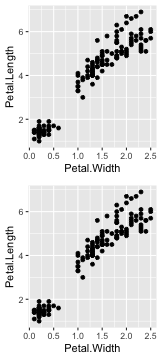This document explains concepts and basics of `{ggfortify}`. `{ggfortify}` helps plotting some popular R packages with `{ggplot2}` in a unified way. See github to check the list of supported packages / classes.

# Loading Package

``````library(ggfortify)
``````

# Using `ggplot2::autoplot`

This is the easiest way provided by `{ggfortify}`. Calling `autoplot` with supported instance should output appropriate plot based on its type, as standard `plot` function does.

``````autoplot(AirPassengers)
``````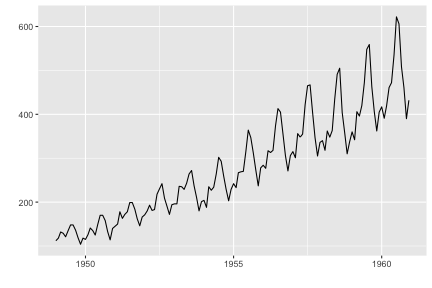You can specify some options to control how plot looks. As is often the case with statistic classes, there are some objects to be drawn, such as actual value, predicted value and confidence interval, etc. Thus, each plotting option has a dot-separated format like `<target name>.<ggplot option name>` such as `ts.colour` and `conf.int.linetype`.

These options can be used for the similar type of instances commonly. For example, `ts.colour` works for all time-series-likes (there is no separate options like `xts.colour` and `timeSeries.colour`, etc). To check available options, use `help(autoplot.ts)` or `help(autoplot.*)` for any other objects.

``````autoplot(AirPassengers, ts.colour = 'blue')
``````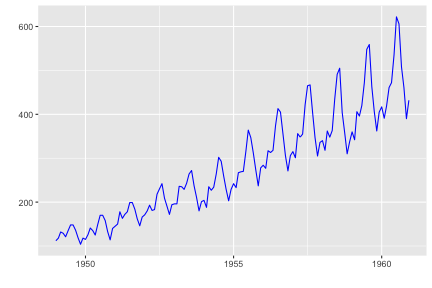# Decorating Plots

`autoplot` returns `ggplot` instance. Thus, you can decorate as the same as in `{ggplot2}`. To make `autoplot` API simple, `{ggfortify}` only offers some limited options which must be done during `ggplot` instance creation. Additional decoration should be done against the returned `ggplot` instance.

``````p <- autoplot(AirPassengers)
class(p)
``````
``````##  "gg"     "ggplot"
``````
``````# plot as it is
p
````````````# add title and labels
p + ggtitle('AirPassengers') + xlab('Year') + ylab('Passengers')
``````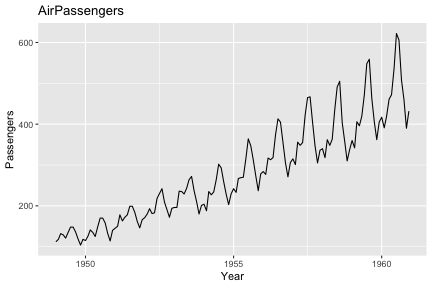``````# these common options are supported as keywords
autoplot(AirPassengers, title = 'AirPassengers', xlab = 'Year', ylab = 'Passengers')
``````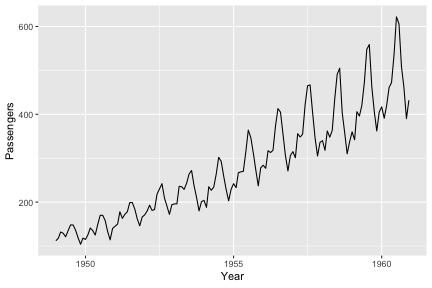``````set.seed(1)
p <- autoplot(kmeans(iris[-5], 3), data = iris)
# plot as it is
p
``````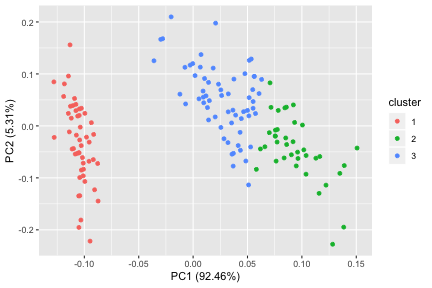``````# change colour mapping
p + scale_colour_brewer()
``````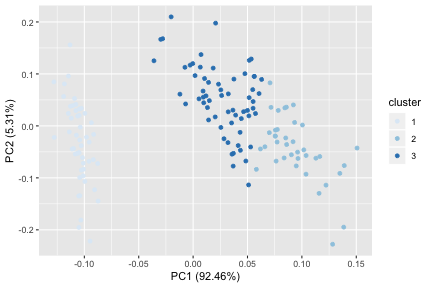# Want Different Plots?

Internally, `autoplot` calls a generic function named `ggplot2::fortify` to convert the passed instance to `data.frame`. `{ggfortify}` defines `fortify` function for all supported classes. If you want a different type of plot, you can use `fortify` to get `data.frame`, then call `ggplot` in a normal way.

Following example shows a bar plot counting records per k-means clusters.

``````df <- fortify(kmeans(iris[-5], 3), data = iris)
head(df)
``````
``````##   Sepal.Length Sepal.Width Petal.Length Petal.Width Species cluster
## 1          5.1         3.5          1.4         0.2  setosa       2
## 2          4.9         3.0          1.4         0.2  setosa       2
## 3          4.7         3.2          1.3         0.2  setosa       2
## 4          4.6         3.1          1.5         0.2  setosa       2
## 5          5.0         3.6          1.4         0.2  setosa       2
## 6          5.4         3.9          1.7         0.4  setosa       2
``````
``````ggplot(df, aes(x= cluster, fill = cluster)) + geom_bar()
``````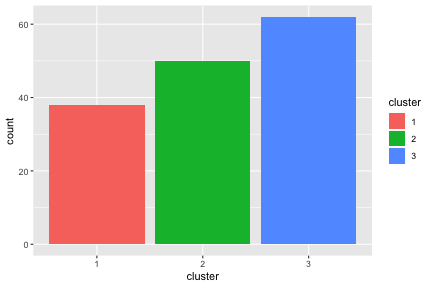# Handling Subplots

`autoplot` may draw subplots in some classes. For exmple, `autoplot.lm` draws diagnostics plots as below.

``````res <- lm(Volume ~ Girth, data = trees)
mp <- autoplot(res, ncol = 4)
mp
``````

Because `{ggplot2}` itself cannot handle different kinds of plots in a single instance, `{ggfortify}` handle them using its original class named `ggmultiplot`. You can use `+` operator to decorate `ggmultiplot`.

``````class(mp)
mp + theme_bw()
``````

Also, `+` operator appends plots if `ggplot` or `ggmultiplot` instance is given as right-hand-side. Following example attaches 2 scatter plots after diagnostics.

``````mp +
(ggplot(trees, aes(Girth, Volume)) + geom_point()) +
(ggplot(trees, aes(Girth, Height)) + geom_point())
``````

You can extract subset using `[` and `[[` operators.

``````mp[2:3]
``````
``````mp[]
``````

You can extract arbitrary subset, modify, and draw.

``````mp[2:3] <- mp[2:3] + theme_bw()
mp
``````

## Draw Multiple Instances as Subplots

Assuming you want to plot multiple instances at once. `{ggfortify}` can accept `list` of supported instances and can output subplots.

Following example shows 3 `kmeans` plots changing number of clusters.

``````res <- lapply(c(3, 4, 5), function(x) kmeans(iris[-5], x))
autoplot(res, data = iris[-5], ncol = 3)
``````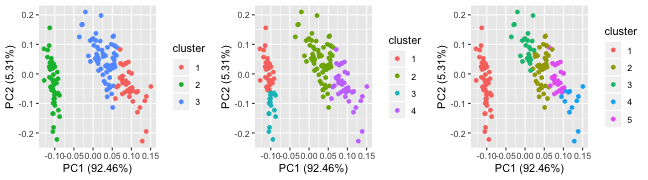Next example shows multiple survival curves as subplots.

``````library(survival)
res <- list(a = survfit(Surv(time, status) ~ 1, data = lung),
b = survfit(Surv(time, status) ~ sex, data = lung))
autoplot(res)
``````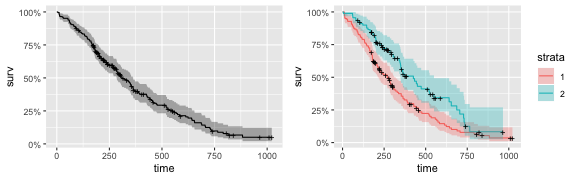You can pass a list contains different classes to `autoplot`.

``````library(vars)
data(Canada)
res <- list(a = Canada, b = AirPassengers)
autoplot(res)
``````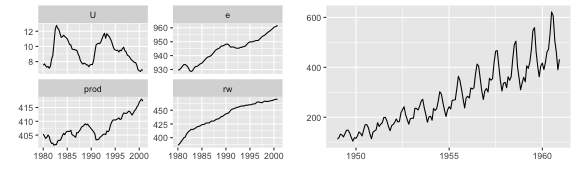## Create your own subplots

You can instanciate `ggmultiplot` from a `list` of `ggplot` instances.

``````p1 <- ggplot(iris, aes(Petal.Width, Petal.Length)) + geom_point()
p2 <- ggplot(iris, aes(Petal.Width, Petal.Length)) + geom_point()
new('ggmultiplot', plots = list(p1, p2))
``````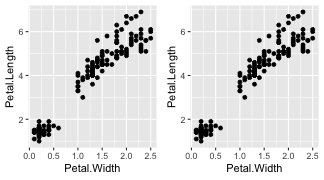Layout can be specified via `nrow` and `ncol` keywords.

``````new('ggmultiplot', plots = list(p1, p2), ncol = 1)
``````